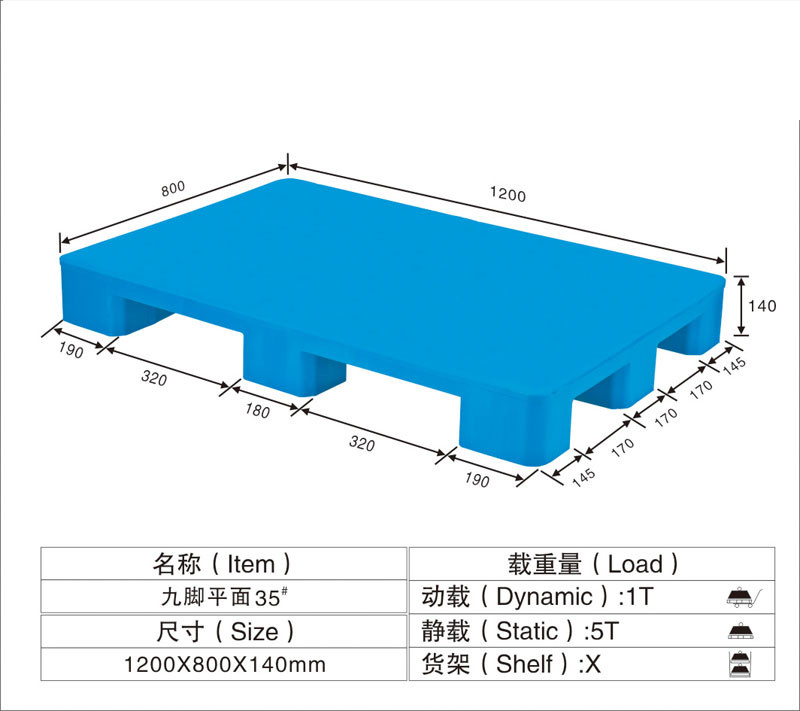QQ：772958196

E-mail：772958196@qq.com1.九角吹塑：

1000×800×145   1000×1000×145   1040×760×145
1054×762×172   1100×900×145   1100×1100×145
1140×1140×140   1200×800×145   1200×1000×140（重型）
1200×1000×1400（轻型） 1200×1200×145   1300×1100×140
2.川字吹塑：

1020×715×160   1100×1100×160   1200×1000×160（A/B）
1200×1200×160   1300×1100×160   1400×1200×160
1500×1200×160
3.田字吹塑：

1100×1100×160   1200×1100×160   1200×1200×160
1300×1100×160   1400×1200×160   1500×1200×160
4.双面吹塑：

1200×1000×150   1200×1200×150   1300×1100×150
1400×1200×150（四面进叉） 1400×1200×150（两面进叉）
1500×1300×150   1500×1500×150   1500×1250×150
1500×1200×150   1500×1400×150   1600×1400×150

1.六角平板：

1000×500×135
2.单面九角网格：

1000×800×145   1000×1000×140   1000×1000×145
1100×800×145    1000×900×145    1100×1000×145
1100×1100×135   1100×1100×140   1100×1100×145（带卡槽）
1100×1100×145   1200×800×145    1200×900×145
1200×1000×110   1200×1000×135   1200×1000×145（带卡槽）
1200×1000×145   1200×1100×145   1200×1200×145（带卡槽）
1300×1100×145
3.单面九角平板：

1100×1100×78   1100×1100×140   1200×800×140
1200×1000×78   1200×1000×140    1200×1000×110
4.单面九角垫板（网格、垫板）

1100×1100×50   1100×1100×78   1200×1000×78
5.单面川字网格：

1100×1100×150   1200×800×170   1200×1000×150
1200×1000×170   1200×1000×170
6.单面川字平板：

1200×1000×150   1200×1000×160
7.单面川字网格加钢管：

1100×1100×160（3钢） 1200×800×145（3钢）
1200×1000×150（3钢） 1200×1000×150（8钢）
1200×1100×150（3钢） 1200×1200×150（8钢）
1300×1100×150（8钢） 1400×1100×170（8钢）
8.单面田字网格：

1100×1100×125   1100×1100×150（负重大） 1100×1100×150（无坎）
1100×1100×150    1200×1000×125    1200×1000×150
1200×1000×150   1200×1000×170   1200×1200×150
1300×1100×170   1000×1000×150
9.单面田字平板：

1100×1100×150
10.单面田字网格加钢管：

1100×1100×150（8钢） 1200×1000×150（8钢） 1200×1000×150（7钢）
11.单面田字平板加钢管：

1100×1100×150（8钢） 1200×1000×150（8钢）
12.双面网格：

1100×1100×150   1200×1000×150   1400×1200×150
13.双面平板：

1100×1100×150   1200×1000×150
14.双面平板加钢管：

1100×1100×150（8钢） 1200×1000×150（8钢）
15.双面网格加钢管：

1100×1100×150（8钢） 1200×1100×150（8钢） 1200×1200×150（8钢）